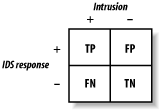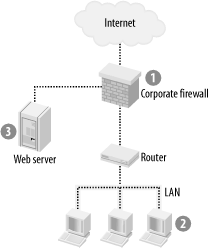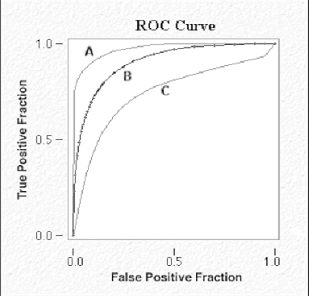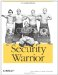# 19.2 Bayesian Analysis

 <  Day Day Up  >

Because of the nature of IDSs, they are always at a disadvantage . Hackers can always engineer new exploits that will not be detected by existing signature databases. In addition, as with virus scanners , keeping signatures up to date is a major problem. Furthermore, network IDSs are expected to cope with massive bandwidth. Maintaining state in a high-traffic network becomes prohibitive in terms of memory and processing cost.

Moreover, monitoring "switched networks" is problematic because switches curtail the IDS's sensors. There have been attempts to compensate for this by embedding the IDS in the switch or attaching the IDS to the switch monitor port. However, such solutions have multiple unresolved challenges. For example, mirroring a set of gigabit links requires deploying multiple IDSs in a complicated load-balancing configuration, since no single IDS is able to cope with the load.

Another limitation of IDSs is that they are extremely vulnerable to attack or evasion. For example, denial-of-service attacks such as SYN floods or smurf attacks can often take down an IDS with ease. Similarly, slow scans or IP address spoofing frustrate many IDSs.

This section introduces the statistical properties of diagnostic tests and their implications for interpreting test results. We use a principle from statistics known as the Bayes's theorem , which describes the relationships that exist within an array of simple and conditional probabilities. Rather than covering the mathematical details, which can be obtained from any of hundreds of statistics books, we instead focus on a practical implementation of "Bayesian analysis" as applied to IDSs. Understanding these concepts and their practical implementation will enable you to make better judgments about how to place different flavors of IDS at different points in your network. 

 This approach to sensor placement evolved from a course on Bayesian diagnosis, taught to medical students by one of the authors.

#### 19.2.1 Sensitivity Versus Specificity

Consider a typical IDS report monitor as represented by the 2 x 2 table in Figure 19-1. One axis, called "Intrusion," represents whether an intrusion has really occurred ”the "+" means there really was an intrusion, while the "-" means there was no intrusion. The other axis, called "IDS Response," represents whether the IDS thinks it has detected an intrusion ”the "+" means the IDS thinks there was an intrusion, while the "-" means the IDS thinks there was no intrusion. As in the real world, this model shows that the IDS is not always correct. We can use the incidence of each quadrant of the 2 x 2 table to help us understand the statistical properties of an IDS.

##### Figure 19-1. IDS response matrixHere's what the initials in the table represent:

TP = true positive (intrusion correctly detected)
FP = false positive (false alarm)
FN = false negative (intrusion missed)
TN = true negative (integrity correctly detected)
##### 19.2.1.1 Sensitivity

Sensitivity is defined as the true-positive rate (i.e., the fraction of intrusions that are detected by the IDS). Mathematically, sensitivity is expressed as follows :

True positives / (true positives + false negatives )

The false-negative rate is equal to 1 minus the sensitivity. The more sensitive an IDS is, the less likely it is to miss actual intrusions.

Sensitive IDSs are useful for identifying attacks on areas of the network that are easy to fix or should never be missed. Sensitive tests are more useful for "screening" ”i.e., when you need to rule out anything that might even remotely possibly represent an intrusion. Among sensitive IDSs, negative results have more inherent value than positive results.

For example, you need a sensitive IDS to monitor host machines sitting deep in the corporate LAN, shielded by firewalls and routers. In Figure 19-2, Area 2 represents this kind of machine. At this heavily buffered point in the network, we should not have any intrusions whatsoever. It is important to have a high level of sensitivity in order to screen for anything amiss. Specificity is less important because at this point in the network, all anomalous behavior should be investigated. The IDS does not need to discriminate, since a human operator is obligated to investigate each alarm by hand.

##### Figure 19-2. Network segmentation for Bayesian optimization of IDS placement##### 19.2.1.2 Specificity

Mathematically, specificity is expressed as follows:

True negatives / (true negatives + false positives)

True negatives represent occasions when the IDS is correctly reporting no intrusions. False positives occur when an IDS mistakenly reports an intrusion when there actually is none. The false-positive rate is equal to 1 minus the specificity.

Specific IDSs have the greatest utility to the network administrator. For these programs, positive results are more useful than negative results. Specific tests are useful when consequences for false-positive results are serious.

Choose an IDS with high specificity for an area of the network in which automatic diagnosis is critical. For example, Area 1 in Figure 19-2 represents a corporate firewall that faces the Internet. In this case, we need an IDS that has a high specificity to detect denial-of-service attacks, since these attacks can be fatal if not detected early. At this point in the network, we care less about overall sensitivity, since we are " ruling in" an attack, rather than screening the mass of normal Internet traffic for any anomalies.

##### 19.2.1.3 Accuracy

Often, the trade-off between sensitivity and specificity varies on a continuum that depends on an arbitrary cutoff point. A cutoff for abnormality may be chosen liberally or conservatively. However, there are situations when we need to spend the extra money to achieve high sensitivity and high specificity. Accuracy is a term that encompasses both specificity and sensitivity. Accuracy is the proportion of all IDS results (positive and negative) that are correct.

For example, we might need high accuracy in an area of the network such as Area 3 in Figure 19-2. In this case, our web server is under constant attack, and it would cause us immediate embarrassment and financial loss if compromised. We need to process any slight anomaly, and we need to do it automatically because of the high traffic volume. In fact, to achieve the highest sensitivity and specificity, we might need to combine layers of different IDSs.

The receiver operating characteristic (ROC) curve is a method of graphically demonstrating the relationship between sensitivity and specificity. An ROC curve plots the true-positive rate (sensitivity) against the false-positive rate (1 minus specificity). This graph serves as a nomogram (Figure 19-3), which is a graphical representation (from the field of statistics) that helps you to quickly compare the quality of two systems.

After choosing a desired cutoff point, the IDS's sensitivity and specificity can be determined from the graph. The curve's shape correlates with the accuracy or overall quality of the IDS. A straight line moving up and to the right at 45 degrees indicates a useless IDS. In contrast, an IDS in which the ROC curve is tucked into the upper left-hand corner of the plot offers the best information. Quantitatively, the area under the curve is correlated directly with the accuracy of the IDS.

In Figure 19-3, the IDS labeled B is more accurate than IDS C. The IDS labeled A has the highest accuracy of all.

##### Figure 19-3. Sample ROC curve#### 19.2.2 Positive and Negative Predictive Values

Theoretically, sensitivity and specificity are properties of the IDS itself; these properties are independent of the network being monitored . Thus, sensitivity and specificity tell us how well the IDS itself performs, but they do not show how well it performs in the context of a particular network. In contrast, predictive value accounts for variations in underlying networks and is more useful in practice.

Predictive values are real-world predictions derived from all available data. Predictive value combines prior probability with IDS results to yield post-test probability, expressed as positive and negative predictive values. This combination constitutes a practical application of Bayes's theorem, which is a formula used in classic probability theory.

Information based on attack prevalence in your network is adjusted by the IDS result to generate a prediction. Most network administrators already perform this analysis intuitively but imprecisely. For example, if you know that slow ping sweeps have recently become prevalent against your network, you use that information to evaluate data from your IDS.

When various predictors are linked mathematically, they must be transformed from probabilities to odds. Then, they are referred to as likelihood ratios (LRs) or odds ratios (ORs) and can be combined through simple multiplication.

#### 19.2.3 Likelihood Ratios

Sensitivity, specificity, and predictive values are all stated in terms of probability : the estimated proportion of time that intrusions occur. Another useful term is odds (i.e., the ratio of two probabilities, ranging from zero [never] to infinity [always]). For example, the odds of 1 are equivalent to a 50% probability of an intrusion (i.e., just as likely to have occurred as not to have occurred). The mathematical relation between these concepts can be expressed as follows:

Odds = probability / (1 - probability)
Probability = odds / (1 + odds)

LRs and ORs are examples of odds. LRs yield a more sophisticated prediction because they employ all available data.

The LR for a positive IDS result is defined as the probability of a positive result in the presence of a true attack, divided by the probability of a positive result in a network not under attack (true-positive rate/false-positive rate). The LR for a negative IDS result is defined as the probability of a negative result in the absence of a true attack, divided by the probability of a negative result in a network that is under attack (true-negative rate/false-negative rate).

LRs enable more information to be extracted from a test than is allowed by simple sensitivity and specificity. When working with LRs and other odds, the post-test probability is obtained by multiplying together all the LRs. The final ratio can also be converted from odds to probability to yield a post-test probability.

By applying these statistical methods , we can make informed choices about deploying IDSs throughout a network. Although currently fraught with inaccuracy, the field of intrusion detection is still nascent, and new and exciting developments are happening every day. As time goes on, use of the scientific method will improve this inexact and complex technology. By understanding the sensitivity and specificity of an IDS, we can learn its value and when to utilize it. In addition, increasing the use of likelihood ratios makes the data that we receive from our IDSs more meaningful.

 <  Day Day Up  >Security Warrior
ISBN: 0596005458
EAN: 2147483647
Year: 2004
Pages: 211

Similar book on Amazon